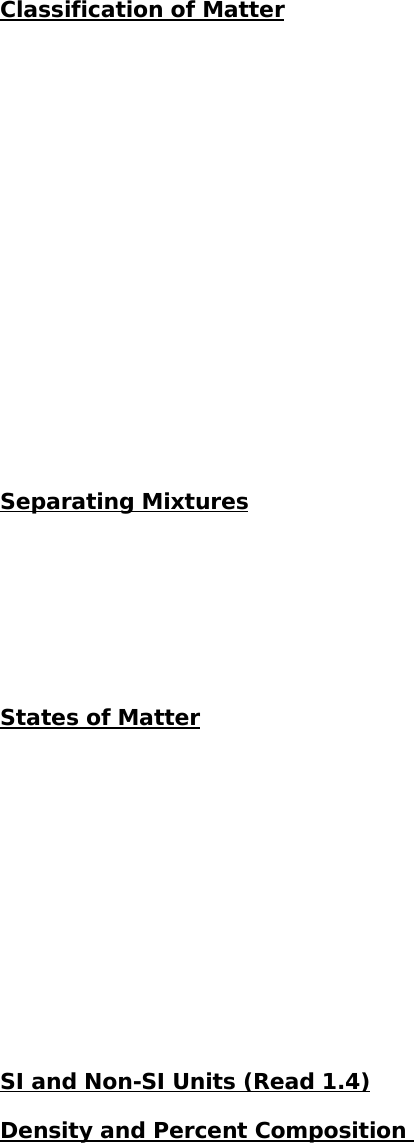Textbook Notes (280,000)
CA (160,000)
UTSG (10,000)
CHM (200)
Chapter 1

CHM426H1 Chapter Notes - Chapter 1: Significant Figures, Decimal Mark, Osmium

Department
Chemistry
Course Code
CHM426H1
Professor
A J Bonner
Chapter
1

Page:
of 4Chemistry Chapter One:
Classification of Matter
Matter is made up of very tiny units called atoms, of which we know about 114 types.
Each type of atom is the building block of a different chemical element, thus there are
114 elements. 90% of all elements are natural and the remaining percentage of
elements is those synthesized in the laboratories.
When two or more elements are joined, they form a compound. A compound is made
of many molecules. A molecule is the smallest entity having the same
proportions of the constituent atoms as does the compound as a whole.
An element and compounds are referred to as substances. When two or more
substances mix, they form a mixture. When a mixture is uniform in composition and
properties throughout, it is known as a homogeneous mixture or solution (e.g.
ordinary air, seawater, and gasoline). When the composition and physical properties
vary from one part of the mixture to another, it is known as a heterogeneous
mixture (e.g. sand and water).
Separating Mixtures
Consider the mixture of sand and water being poured into a funnel lined with porous
filter paper. The water passes through, but the sand is retained. This process of
separation is known as filtration. On the other hand, we cannot filter a
homogeneous mixture of copper (II) sulphate. However, this compound can be
separated by boiling. This process is called distillation.
States of Matter
Matter generally exists in 3 states: solid, liquid, or gas. A solid contains atoms or
molecules in close contact, sometimes in a highly organized arrangement called a
crystal. As well, a solid had a definite shape. In a liquid, the atoms or molecules are
usually further apart. The movement of these atoms/molecules give a liquid its most
distinctive property: the ability to flow, covering the bottom and assume the
shape of its container. In a gas, the distance between atoms/ molecules is much
greater. A gas always expands to fill its container. ***In water, specifically ice, it is
important to remember that liquid water is more dense than ice, since all the
molecules in the liquid are joined tightly to each other to provide a sturdy framework
for a block of ice.***
SI and Non-SI Units (Read 1.4)
Density and Percent Composition
When someone says a ton of bricks weigh more than a ton of cotton, they have the
concepts of weight and density confused. Matter in a brick is more concentrated
than in cotton, making bricks denser than cotton. Density is the ration of mass to
volume. Density (d) = mass (m)/ volume (v). Mass and volume are extensive
properties. An extensive property is dependent on the quantity of matter observed,
unless, the mass of the substance is divided by its volume. In this case, that is an
intensive property. This is an independent of the amount of matter observed. (e.g.
the density of water at 25ºC is the same whether it fills a small beaker or a large pool.
Density is a function of temperature because volume varies with temperature,
whereas mass remains constant. For example, as the average temperature of
seawater increases, the seawater will become less dense and its volume will increase.
Another factor that affects density is state of matter. Generally, solids are denser
than liquids, and both are denser than gases.
Density in Conversion Pathways
1. A cube of osmium 1.000 cm on edge weighs 22.48g. The density of osmium,
therefore, is 22.48g/cm3. What would be the mass of a cube of osmium that is
1.25 in on edge (1 in = 2.54cm)?
To solve this, we need to remember that V= l3.
? g osmium = [ 1.25 in. x (2.54 cm/ 1 in.)] 3x (22.48 g osmium/ 1 cm3) = 719 g
osmium
2. At 25ºC the density of mercury is 13.5 g/mL. What is the volume, in mL, of
1.000 kg of mercury at 25ºC?
? mL mercury = 1.000 kg x (1000 g/ 1 kg) x (1 mL mercury/ 13.5 g) = 74.1 mL
mercury
(Also see example 1.2 and 1.3)
Percent Composition
1. Currently, U.S. pennies are 2.40% copper and 97.60% zinc. What mass of each
element is in a penny weighing 2.485g?
Consider 100 g of penny. Copper is (2.40 g copper/ 100 g penny) and zinc is
(97.60g zinc/ 100g penny).
(2.40 g copper/ 100 g penny) x 2.485 g penny = 0.0596 g copper
(97.60 g zinc/ 100 g penny) x 2.485 g penny = 2.425 g zinc
2. A 75-g sample of sodium chloride is to be produced by evaporating to dryness
a quantity of seawater containing 3.5 % sodium chloride by mass. How many
liters of seawater must be taken for this purpose? Assume a density of 1.03 g/
mL for seawater.
? L seawater = 75 g sodium chloride x (100 g seawater / 3.5 g sodium
chloride) x (1 mL seawater / 1.03 g seawater) x (1 L seawater / 1000 mL
seawater)
= 2.1 L seawater
Uncertainties in Scientific Measurement
Precision refers to how well a number or independent measurement agrees with one
another. Accuracy refers to how close to the true value a given measurement is
(e.g. a bathroom scale is neither accurate nor precise, a lab balance is fairly accurate
but not very precise, but an analytical balance is both accurate and precise).
Significant Figures
Consider:
0. 004004500
Rounding Rules
1. If the first digit you remove is less than 5, round down by dropping it and all
following digits.
Example: 5.664 525 = 5.66
Not
significant:
zero used
for
cosmetic
Not significant:
zeros used only
to locate the
decimal point.
Significant: all
non-zeros
integers
Significant: all
zeros between
Significant: zeros
at the end of a
number to the
right of decimal
point.